# 4.1 Linear functions  (Page 16/27)

 Page 16 / 27

Write an equation for a line perpendicular to $\text{\hspace{0.17em}}h\left(t\right)=-2t+4\text{\hspace{0.17em}}$ and passing through the point $\text{\hspace{0.17em}}\left(-4,–1\right).$

Write an equation for a line perpendicular to $\text{\hspace{0.17em}}p\left(t\right)=3t+4\text{\hspace{0.17em}}$ and passing through the point $\text{\hspace{0.17em}}\left(3,1\right).$

$y=-\frac{1}{3}t+2$

## Graphical

For the following exercises, find the slope of the line graphed.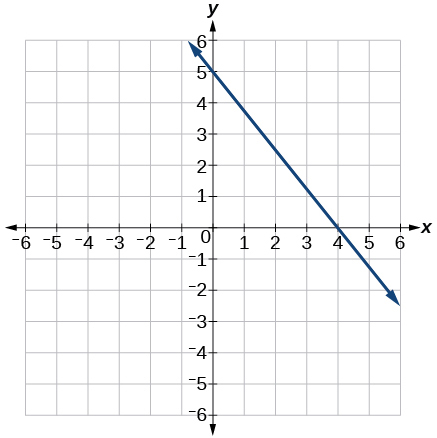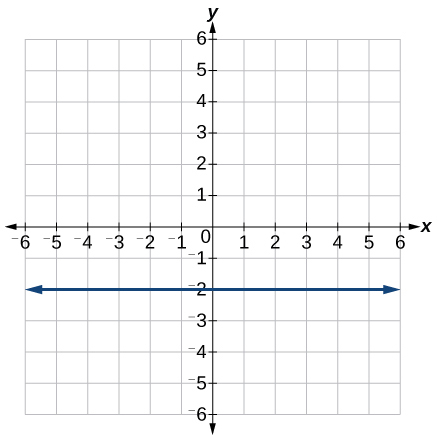0

For the following exercises, write an equation for the line graphed.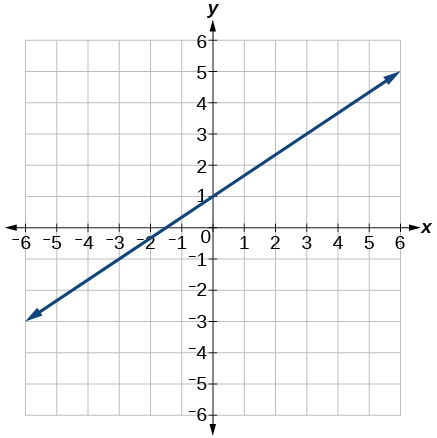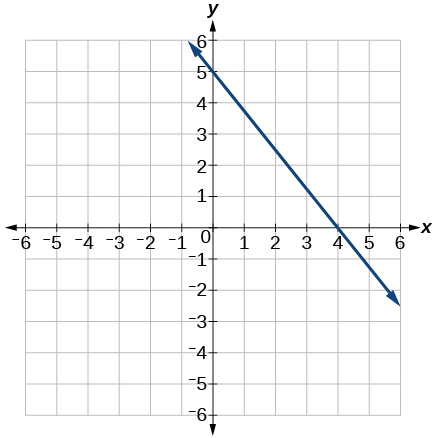$y=-\frac{5}{4}x+5$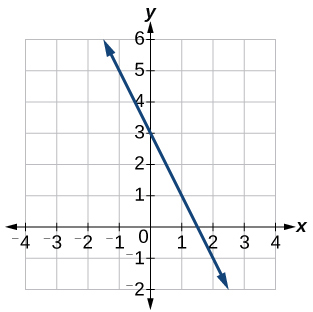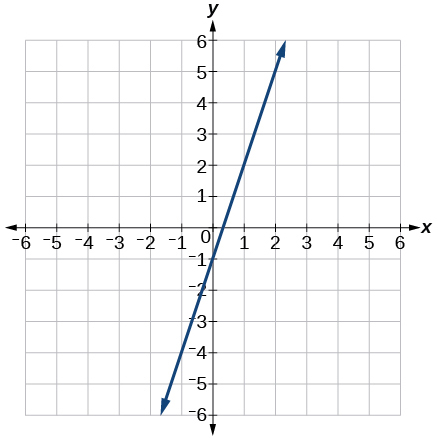$y=3x-1$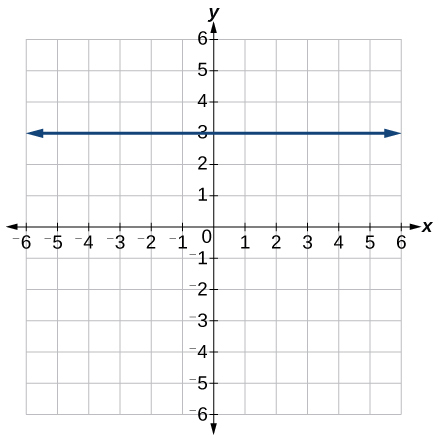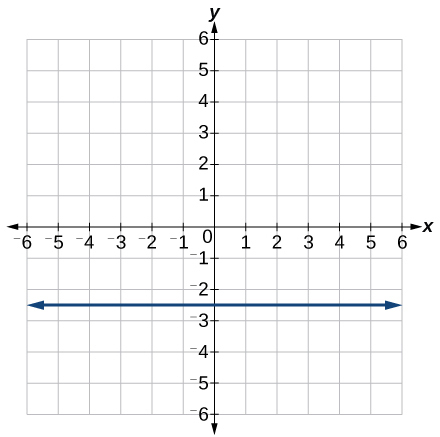$y=-2.5$

For the following exercises, match the given linear equation with its graph in [link] .

$f\left(x\right)=-x-1$

$f\left(x\right)=-2x-1$

F

$f\left(x\right)=-\frac{1}{2}x-1$

$f\left(x\right)=2$

C

$f\left(x\right)=2+x$

$f\left(x\right)=3x+2$

A

For the following exercises, sketch a line with the given features.

An x -intercept of $\text{\hspace{0.17em}}\left(–4,\text{0}\right)\text{\hspace{0.17em}}$ and y -intercept of $\text{\hspace{0.17em}}\left(0,\text{–2}\right)$

An x -intercept $\text{\hspace{0.17em}}\left(–2,\text{0}\right)\text{\hspace{0.17em}}$ and y -intercept of $\text{\hspace{0.17em}}\left(0,\text{4}\right)$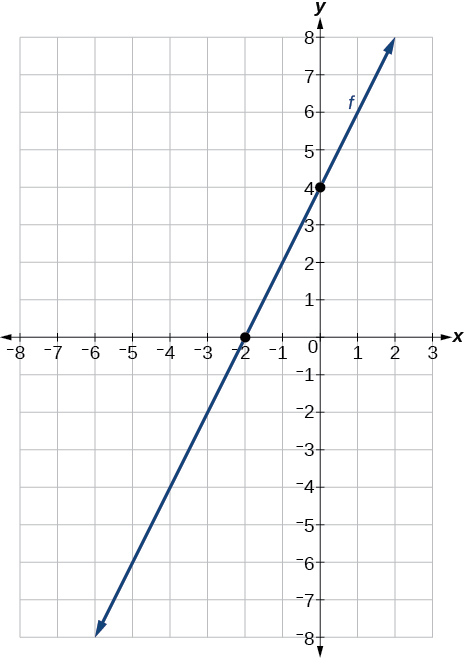A y -intercept of $\text{\hspace{0.17em}}\left(0,\text{7}\right)\text{\hspace{0.17em}}$ and slope $\text{\hspace{0.17em}}-\frac{3}{2}$

A y -intercept of $\text{\hspace{0.17em}}\left(0,\text{3}\right)\text{\hspace{0.17em}}$ and slope $\text{\hspace{0.17em}}\frac{2}{5}$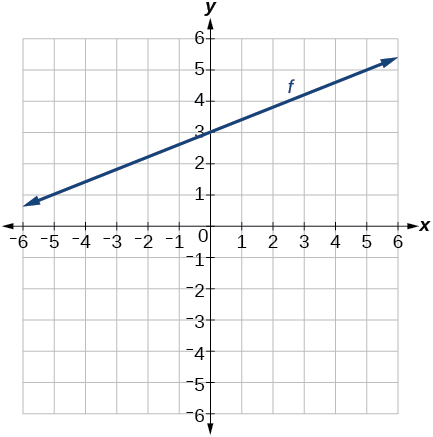Passing through the points $\text{\hspace{0.17em}}\left(–6,\text{–2}\right)\text{\hspace{0.17em}}$ and $\text{\hspace{0.17em}}\left(6,\text{–6}\right)$

Passing through the points $\text{\hspace{0.17em}}\left(–3,\text{–4}\right)\text{\hspace{0.17em}}$ and $\text{\hspace{0.17em}}\left(3,\text{0}\right)$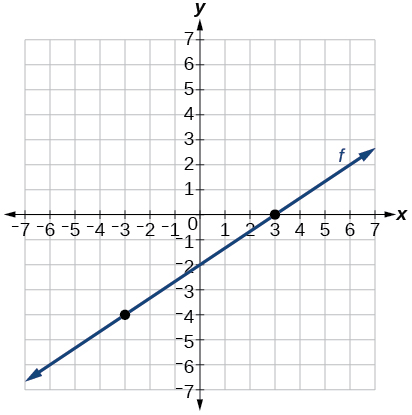For the following exercises, sketch the graph of each equation.

$f\left(x\right)=-2x-1$

$f\left(x\right)=-3x+2$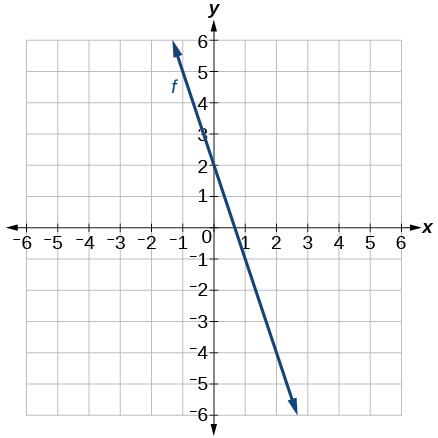$f\left(x\right)=\frac{1}{3}x+2$

$f\left(x\right)=\frac{2}{3}x-3$$f\left(t\right)=3+2t$

$p\left(t\right)=-2+3t$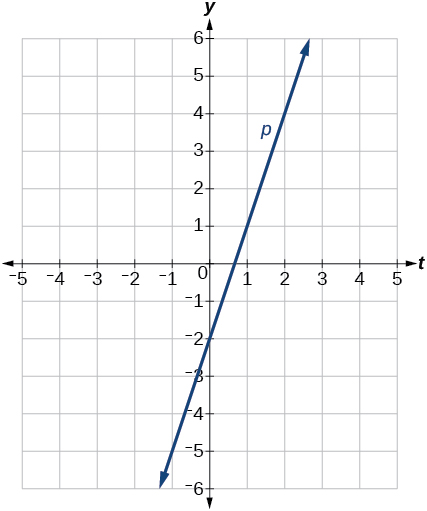$x=3$

$x=-2$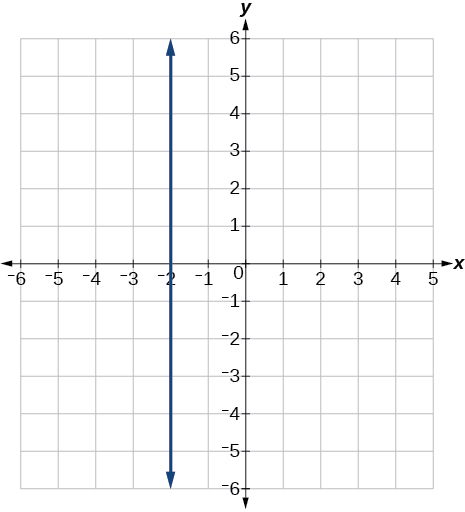$r\left(x\right)=4$

For the following exercises, write the equation of the line shown in the graph.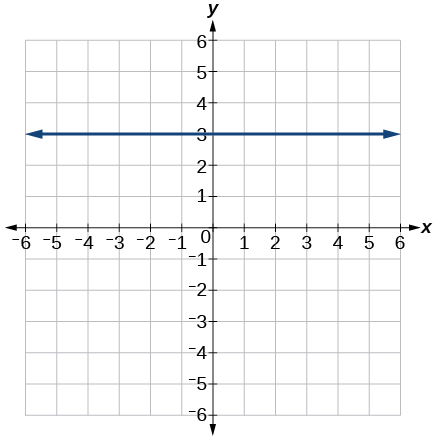$y=\text{3}$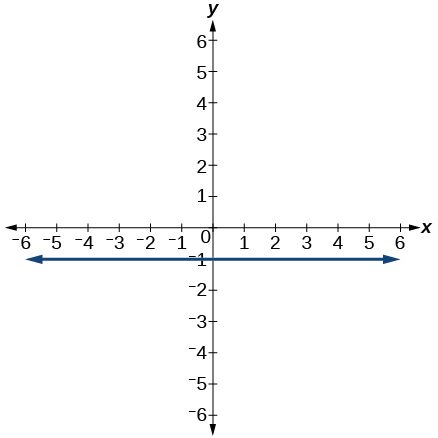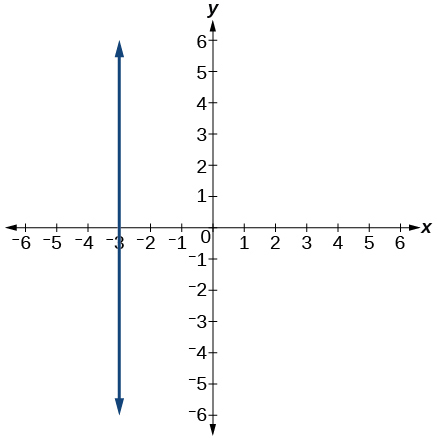$x=-3$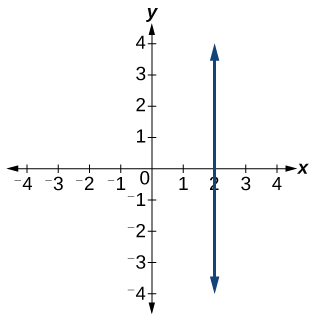## Numeric

For the following exercises, which of the tables could represent a linear function? For each that could be linear, find a linear equation that models the data.

 $x$ 0 5 10 15 $g\left(x\right)$ 5 –10 –25 –40

Linear, $\text{\hspace{0.17em}}g\left(x\right)=-3x+5$

 $x$ 0 5 10 15 $h\left(x\right)$ 5 30 105 230
 $x$ 0 5 10 15 $f\left(x\right)$ –5 20 45 70

Linear, $\text{\hspace{0.17em}}f\left(x\right)=5x-5$

 $x$ 5 10 20 25 $k\left(x\right)$ 13 28 58 73
 $x$ 0 2 4 6 $g\left(x\right)$ 6 –19 –44 –69

Linear, $\text{\hspace{0.17em}}g\left(x\right)=-\frac{25}{2}x+6$

 $x$ 2 4 8 10 $h\left(x\right)$ 13 23 43 53
 $x$ 2 4 6 8 $f\left(x\right)$ –4 16 36 56

Linear, $\text{\hspace{0.17em}}f\left(x\right)=10x-24$

 $x$ 0 2 6 8 $k\left(x\right)$ 6 31 106 231

## Technology

For the following exercises, use a calculator or graphing technology to complete the task.

If $\text{\hspace{0.17em}}f\text{\hspace{0.17em}}$ is a linear function, find an equation for the function.

$f\left(x\right)=-58x+17.3$

Graph the function $\text{\hspace{0.17em}}f\text{\hspace{0.17em}}$ on a domain of $\text{\hspace{0.17em}}\left[–10,10\right]:f\left(x\right)=0.02x-0.01.\text{\hspace{0.17em}}$ Enter the function in a graphing utility. For the viewing window, set the minimum value of $\text{\hspace{0.17em}}x\text{\hspace{0.17em}}$ to be $\text{\hspace{0.17em}}-10\text{\hspace{0.17em}}$ and the maximum value of $\text{\hspace{0.17em}}x\text{\hspace{0.17em}}$ to be $\text{\hspace{0.17em}}10.$

Graph the function $\text{\hspace{0.17em}}f\text{\hspace{0.17em}}$ on a domain of $\text{\hspace{0.17em}}\left[–10,10\right]:fx\right)=2,500x+4,000$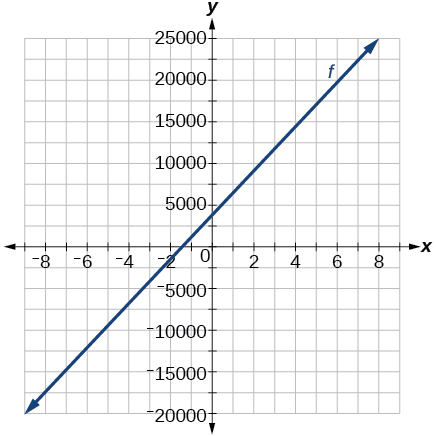[link] shows the input, $\text{\hspace{0.17em}}w,$ and output, $\text{\hspace{0.17em}}k,$ for a linear function $\text{\hspace{0.17em}}k.\text{\hspace{0.17em}}$ a. Fill in the missing values of the table. b. Write the linear function $\text{\hspace{0.17em}}k,$ round to 3 decimal places.

 w –10 5.5 67.5 b k 30 –26 a –44

[link] shows the input, $\text{\hspace{0.17em}}p,$ and output, $\text{\hspace{0.17em}}q,$ for a linear function $\text{\hspace{0.17em}}q.\text{\hspace{0.17em}}$ a. Fill in the missing values of the table. b. Write the linear function $\text{\hspace{0.17em}}k.$

 p 0.5 0.8 12 b q 400 700 a 1,000,000

Graph the linear function $\text{\hspace{0.17em}}f\text{\hspace{0.17em}}$ on a domain of $\text{\hspace{0.17em}}\left[-10,10\right]\text{\hspace{0.17em}}$ for the function whose slope is $\text{\hspace{0.17em}}\frac{1}{8}\text{\hspace{0.17em}}$ and y -intercept is $\text{\hspace{0.17em}}\frac{31}{16}.\text{\hspace{0.17em}}$ Label the points for the input values of $\text{\hspace{0.17em}}-10\text{\hspace{0.17em}}$ and $\text{\hspace{0.17em}}10.$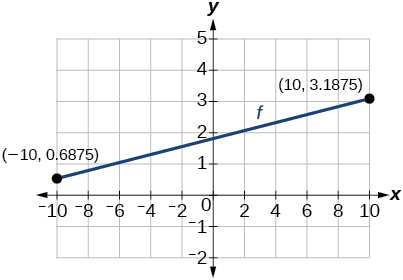Graph the linear function $\text{\hspace{0.17em}}f\text{\hspace{0.17em}}$ on a domain of $\text{\hspace{0.17em}}\left[-0.1,0.1\right]\text{\hspace{0.17em}}$ for the function whose slope is 75 and y -intercept is $\text{\hspace{0.17em}}-22.5.\text{\hspace{0.17em}}$ Label the points for the input values of $\text{\hspace{0.17em}}-0.1\text{\hspace{0.17em}}$ and $\text{\hspace{0.17em}}0.1.$

Graph the linear function $\text{\hspace{0.17em}}f\text{\hspace{0.17em}}$ where $\text{\hspace{0.17em}}f\left(x\right)=ax+b\text{\hspace{0.17em}}$ on the same set of axes on a domain of $\text{\hspace{0.17em}}\left[-4,4\right]\text{\hspace{0.17em}}$ for the following values of $\text{\hspace{0.17em}}a\text{\hspace{0.17em}}$ and $\text{\hspace{0.17em}}b.$

1. $a=2;b=3$
2. $a=2;b=4$
3. $a=2;b=–4$
4. $a=2;b=–5$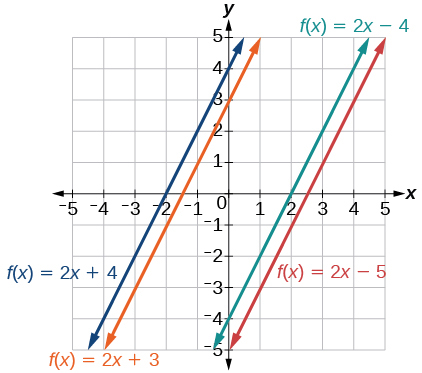## Extensions

Find the value of $\text{\hspace{0.17em}}x\text{\hspace{0.17em}}$ if a linear function goes through the following points and has the following slope: $\text{\hspace{0.17em}}\left(x,2\right),\left(-4,6\right),\text{\hspace{0.17em}}m=3$

Find the value of y if a linear function goes through the following points and has the following slope: $\text{\hspace{0.17em}}\left(10,y\right),\left(25,100\right),\text{\hspace{0.17em}}m=-5$

y = 175

f(x)=x/x+2 given g(x)=1+2x/1-x show that gf(x)=1+2x/3
proof
AUSTINE
sebd me some questions about anything ill solve for yall
how to solve x²=2x+8 factorization?
x=2x+8 x-2x=2x+8-2x x-2x=8 -x=8 -x/-1=8/-1 x=-8 prove: if x=-8 -8=2(-8)+8 -8=-16+8 -8=-8 (PROVEN)
Manifoldee
x=2x+8
Manifoldee
×=2x-8 minus both sides by 2x
Manifoldee
so, x-2x=2x+8-2x
Manifoldee
then cancel out 2x and -2x, cuz 2x-2x is obviously zero
Manifoldee
so it would be like this: x-2x=8
Manifoldee
then we all know that beside the variable is a number (1): (1)x-2x=8
Manifoldee
so we will going to minus that 1-2=-1
Manifoldee
so it would be -x=8
Manifoldee
so next step is to cancel out negative number beside x so we get positive x
Manifoldee
so by doing it you need to divide both side by -1 so it would be like this: (-1x/-1)=(8/-1)
Manifoldee
so -1/-1=1
Manifoldee
so x=-8
Manifoldee
Manifoldee
so we should prove it
Manifoldee
x=2x+8 x-2x=8 -x=8 x=-8 by mantu from India
mantu
lol i just saw its x²
Manifoldee
x²=2x-8 x²-2x=8 -x²=8 x²=-8 square root(x²)=square root(-8) x=sq. root(-8)
Manifoldee
I mean x²=2x+8 by factorization method
Kristof
I think x=-2 or x=4
Kristof
x= 2x+8 ×=8-2x - 2x + x = 8 - x = 8 both sides divided - 1 -×/-1 = 8/-1 × = - 8 //// from somalia
Mohamed
hii
Amit
how are you
Dorbor
well
Biswajit
can u tell me concepts
Gaurav
Find the possible value of 8.5 using moivre's theorem
which of these functions is not uniformly cintinuous on (0, 1)? sinx
which of these functions is not uniformly continuous on 0,1
solve this equation by completing the square 3x-4x-7=0
X=7
Muustapha
=7
mantu
x=7
mantu
3x-4x-7=0 -x=7 x=-7
Kr
x=-7
mantu
9x-16x-49=0 -7x=49 -x=7 x=7
mantu
what's the formula
Modress
-x=7
Modress
new member
siame
what is trigonometry
deals with circles, angles, and triangles. Usually in the form of Soh cah toa or sine, cosine, and tangent
Thomas
solve for me this equational y=2-x
what are you solving for
Alex
solve x
Rubben
you would move everything to the other side leaving x by itself. subtract 2 and divide -1.
Nikki
then I got x=-2
Rubben
it will b -y+2=x
Alex
goodness. I'm sorry. I will let Alex take the wheel.
Nikki
ouky thanks braa
Rubben
I think he drive me safe
Rubben
how to get 8 trigonometric function of tanA=0.5, given SinA=5/13? Can you help me?m
More example of algebra and trigo
What is Indices
If one side only of a triangle is given is it possible to solve for the unkown two sides?
cool
Rubben
kya
Khushnama
please I need help in maths
Okey tell me, what's your problem is?
NavinByByByBy Gerr ZenByByByByBy